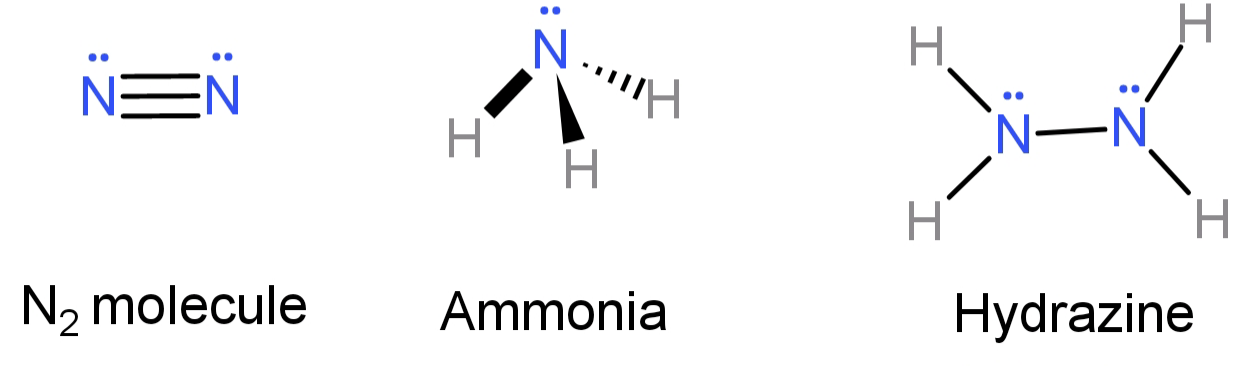Ammonia, $N{H_3}$ and hydrazine, $N{H_2}N{H_2}$, are two compounds of nitrogen, ${N_2}$. Which statement is correct?A.The N-N bond in $N{H_2} - N{H_2}$ is polar.B.$N{H_3}$ and $N{H_2}N{H_2}$ have lone pairs of electrons but ${N_2}$ does not. C.The oxidation number of each nitrogen in $N{H_2}N{H_2}$ is +2.D. The reaction of nitrogen with hydrogen has a high activation rate.Verified
145.5k+ views
Hint: The N-H bond is strong due to the high electronegativity of N atoms and so a large amount of energy is required to break the bond (approximately 386 kJ/mol). Now try all the options and see which one is correct.

-In$N{H_2}N{H_2}$, since both the nitrogen atoms have the same value of electronegativity the bond between the 2 nitrogen atoms (N-N) will be non-polar. This contradicts option (A), so option (A) is false.
-In $N{H_3}$, out of the 5 electrons from the outermost shell of N, 3 have bonded with 1 Hydrogen atom each. Hence leaving behind a lone pair of electrons.
In $N{H_2} - N{H_2}$, for each nitrogen atom having 5 outer shell electrons, 2 are bonded with 1 hydrogen each and with one electron they bond with an electron from the other nitrogen. Hence each of the nitrogen is left with a lone pair of electrons.
In${N_2}$, the 2 N atoms are bonded to each other by a triple bond and so leaving behind a lone pair of electrons each.Hence we now know that all three of them have lone pairs of electrons. This conclusion contradicts option (B), so we can say that option (B) is false.
-In $N{H_2}N{H_2}$: to calculate the oxidation state (O.s.) of N atoms let us assume it to be ‘x’ and the oxidation state of H is already equal to 1. So:
O.s. of 2 N + O.s. of 4 H = O.s. of entire molecule (Total oxidation state of the molecule is 0 because the molecule is electrically neutral
2x + 4 = 0 and so x = -2
Hence the oxidation state of each nitrogen here is (-2). This contradicts option (C), so option (C) is also false.
-The reaction of nitrogen with hydrogen has a high activation rate due to the high bond energy between them (which was due to high electronegativity of N).

Hence, the correct option is: (D).

Note:
Nitrogen reacts with hydrogen only under high temperature and pressure, that too in the presence of a metal catalyst to overcome the high activation energy. It is a type of combination reaction and produces ammonia. This process is also known as Haber’s process.